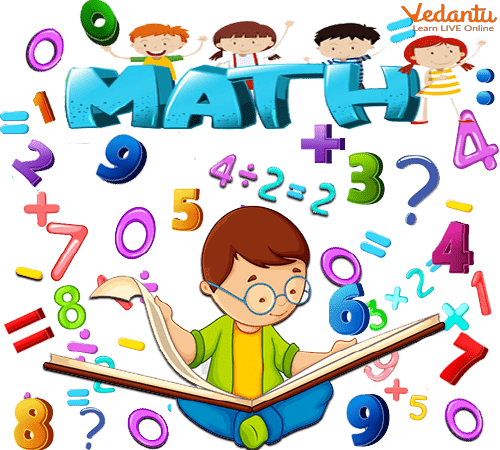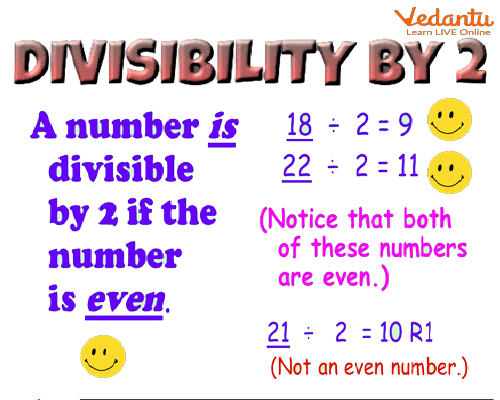Courses
Courses for Kids
Free study material
Free LIVE classes
More

# The Divisibility Rule of 2LIVE
Join Vedantu’s FREE Mastercalss

## What is division?

One of the fundamental operations in mathematics is division. When something is divided, it refers to its separation or sharing into equal portions. The division is the inverse operation of multiplication, just as subtraction is the opposite operation of addition. The slash (/), the horizontal line (-), or the division sign ( ÷ ) can all be used to represent division.

## Divisibility Criteria

We use divisibility criteria to determine whether or not a number is divisible by another. In each case, we apply a different criterion to verify divisibility.Divisibility Rules

Divisibility criteria make it easy to solve a very large number of divisions.

Divisibility criteria are methods used to check whether or not a number is divisible by another. For different numbers, we use different criteria. For example, For 2 divisibility rule, the number just needs to be even, to be divisible by 5, the number must have a unity of 5 or 0. There are different divisibility criteria for each number between 2 and 10.

## Divisibility by 2

The criterion of divisibility test of 2. Understanding what are the criteria for determining the numbers that are divisible by 2.Divisibility by 2

Identifying a number that is a divisibility of 2 is quite easy. Let's analyse this number: it is the first of the prime numbers and it is the only prime number that is even.

At the beginning of the study of numerical sets, the teacher teaches us that an even number can always be written as twice another number. Let's look at numbers divisible by 2 examples:

26 = 2 × 13
28 = 2 × 14
36 = 2 × 18
100 = 2 × 50

Given this fact, we can say that for numbers divisible by 2, it is enough that it be an even number.

But how do you identify an even number? If we answer this question, we will be determining the conditions for identifying a number divisible by 2. However, this is an answer that everyone knows, it is something that we learned in our first contact with numerical sets.

“ Even number is one whose last digit is an even number ”. That is, they are the numbers ending in 0, 2, 4, 6, and 8.

Therefore, the criterion of divisibility by the number 2 occurs in observing if the last digit is even.

However, the number two will not divide all numbers, so it is important to understand the rest of the divisibility test of 2.

Some divisible by 2 examples:

13 0 → is divisible by 2, as it ends in 0;

540 6 → is divisible by 2 as it ends in 6;

123 1 → is not divisible by 2, as it ends in 1;

1,293.02 3 → is not divisible by 2 as it ends in 3.

## Solved Examples

Q1. Check if 268 is divisible by 2.

Ans: Given, 288 is a number.

If the last digit of 268 is 8 which is divisible by 2, then 288 is also divisible by 2.

The last digit of 268 is 8, which is divisible by 2, such that;

8/2 = 4

Hence, 268 satisfies the divisibility of 2 .

Q2. Is 780 divisible by 2?

Ans: Yes, 780 is divisible by 2 as the unit's place digit is 0.

Q1. Check if 197 is divisible by 2 or not.

Ans: 197 is not divisible by 2.

Q2. Which of the following integers is divisible by two, and why?

14    77        46    80        99    26        33    48

Ans: 14 is divisible by 2 as the last digit is an even number.

77 is not divisible by 2 as the last digit is an odd number.

46 is divisible by 2 as the digit in the unit’s place is 6.

80 is divisible by 2 as the last digit is zero.

99 is not divisible by 2 as the last digit is an odd number.

26 is divisible by 2 as the digit at the unit's place is an even.

33 is not divisible by 2 as the number ends with an odd number.

48 is divisible by 2 as the last digit is an even number.

## Summary

The numbers 0, 2, 4, 6, and 8 may all be divided by two. Consequently, multiples of two and integers that finish with 0 are divisible by two. We are aware that even numbers are those that are multiples of 2. As a result, we may state that any even integer is divisible by two.

Numbers like 1, 3, 5, 7, and 9 that end in an odd number cannot be divided by 2.

Last updated date: 01st Oct 2023
Total views: 125.1k
Views today: 2.25k

## FAQs on The Divisibility Rule of 2

1. What is meant by divisibility rules?

A simple technique to determine if a given number may be divided by a set divisor without carrying out the division operation is to run a divisibility test. When a number is fully divided by another number, the remainder should equal zero and the quotient should be a whole number. We may use it to find multiples and factors of numbers without having to perform lengthy divisions. One can determine if an integer is divisible by another integer by utilising the rules for divisibility.

2. What is the 2 divisibility rule?

It has been suggested to use Divisibility criteria or tests to simplify and speed up the division process. Students can more effectively answer the questions if they understand the mathematical division principles and the tests for the divisibility of numbers from 1 to 20.

Rule of numbers divisible by 2: The given integer's last digit must be an even number or a multiple of two, i.e., 0, 2, 4, 6, and 8. For example numbers 100, 224, 1256,1968 are divisible by 2.